Click the “chat” button below for chat support from the developer who created it, or find similar developers for support.CyanideCN228 Stars121 ForksGNU General Public License v3.0408 Commits4 Opened issues#### Description!
?

#### Need anything else?#### Contributors list

`example`
folder contains detailed examples!

## Installation

PyCINRAD supports Python version 3.5 and higher.

```pip install cinrad
```

```python setup.py install
```

## Modules

```from cinrad.io import CinradReader, StandardData
f = StandardData(your_radar_file) #New standard data
f.get_data(tilt, drange, dtype) #Get data
f.get_raw(tilt, drange, dtype)
```

The

`get_raw`
method returns radar records without other geographic information.

The

`get_data`
method returns
`xarray.Dataset`
with radar records, geographic coordinates, and all extra attributes. So, all benefits of
`xarray`
can be enjoyed.
```>>> print(data)

Dimensions:    (azimuth: 366, distance: 920)
Coordinates:
* azimuth    (azimuth) float32 0.14084807 0.15812683 ... 0.12601277 0.14381513
* distance   (distance) float64 0.25 0.5 0.75 1.0 ... 229.2 229.5 229.8 230.0
Data variables:
ZDR        (azimuth, distance) float64 nan nan nan nan ... nan nan nan nan
longitude  (azimuth, distance) float64 120.2 120.2 120.2 ... 120.6 120.6
latitude   (azimuth, distance) float64 35.99 35.99 36.0 ... 38.04 38.04
height     (azimuth, distance) float64 0.1771 0.1792 0.1814 ... 5.218 5.227
Attributes:
elevation:        0.48339844
range:            230
scan_time:        2020-05-17 11:00:28
site_code:        Z9532
site_name:        青岛
site_longitude:   120.23028
site_latitude:    35.98861
tangential_reso:  0.25
nyquist_vel:      8.37801
```

For example, it's very convenient to save data as netcdf format. ```python

data.to_netcdf('1.nc') ```

`xarray`
also makes interpolation very convenient. ```python

data.interp(azimuth=np.deg2rad(300), distance=180) Dimensions: () Coordinates: azimuth float64 5.236 distance int32 180 Data variables: ZDR float64 0.3553 longitude float64 118.5 latitude float64 36.8 height float64 3.6 Attributes: elevation: 0.48339844 range: 230 scantime: 2020-05-17 11:00:28 sitecode: Z9532 sitename: 青岛 sitelongitude: 120.23028 sitelatitude: 35.98861 tangentialreso: 0.25 nyquist_vel: 8.37801 task: VCP21D ```

For single-tilt data (i.e. files that contain only one elevation angle),

`cinrad.io.StandardData.merge`
can merge these files to a file contains full volumetric scan.

#### Export data to `Py-ART` defined class

Convert data structure defined in this module into

`pyart.core.Radar`
is very simple.
`cinrad.io.export`
has a function
`standard_data_to_pyart`
, which can take
`cinrad.io.StandardData`
as input and return
`pyart.core.Radar`
as output.

`example`

#### Decode PUP data and SWAN data

`cinrad.io.PUP`
provides functions to decode PUP data. The extracted data can be further used to create PPI.

`cinrad.io.SWAN`
provides similar interface to decode SWAN data.
```from cinrad.io import PUP
data = f.get_data()
```

#### Decode phased array radar data

`cinrad.io.PhasedArrayData`
provides similar interface to decode level 2 data from phased array radar.
```from cinrad.io import PhasedArrayData
data = f.get_data(0, 40, 'REF')
```

This submodule provides some useful algorithms in radar meteorology. All functions only accept

`numpy.ndarray`
as input data. This submodule extends the usage of this program, as these functions can accept customized data rather than only the data decoded by
`cinrad.io`
.

For direct computation of decoded data,

`cinrad.calc`
provides functions that simplify the process of calculation. For functions contained in this submodule, only a list of reflectivity data is required as the argument.

Code to generate the required list:

```r_list = [f.get_data(i, 230, 'REF') for i in f.angleindex_r]
# or
r_list = list(f.iter_tilt(230, 'REF'))
```

#### VCS

`cinrad.calc.VCS`
provides calculation of vertical cross-section for all variables.
```import cinrad
rl = [f.get_data(i, 230, 'REF') for i in f.angleindex_r]
sec = vcs.get_section(start_cart=(111, 25.5), end_cart=(112, 26.7)) # pass geographic coordinates (longitude, latitude)
sec = vcs.get_section(start_polar=(115, 350), end_polar=(130, 30)) # pass polar coordinates (distance, azimuth)
fig = Section(sec)
fig('D:\\')
```

`cinrad.calc.GridMapper`
can merge different radar scans into a cartesian grid.

#### Hydrometeor classification

`cinrad.calc.hydro_class`
uses algorithm suggested by Dolan to classify hydrometeors into 10 categories. (Requires REF, ZDR, RHO, and KDP)

This submodule provides algorithms to correct raw radar fields.

This function can unwrap the folded velocity using algorithm originated from

`pyart`
. (needs C compiler)
```import cinrad
#(some codes omitted)
v = f.get_data(1, 230, 'VEL')
```

Visualize the data stored in acceptable format (

`cinrad.datastruct`
). It also means that you can using customized data to perform visualization, as long as the data is stored as
`xarray.Dataset`
and constructed by the same protocol (variables naming conventions, data coordinates and dimensions, etc.) For further information about this method, please see the examples contained in
`example`
folder.
```from cinrad.visualize import PPI
fig = PPI(R) #Plot PPI
fig('D:\\') #Pass the path to save the fig
fig = Section(Slice_) #Plot VCS
fig('D:\\')
```

The path passed into the class can either be the folder path or the file path. Also, if no path is passed, the figure will be saved at the folder named

`PyCINRAD`
in the home folder (e.g.
`C:\Users\tom`
).

#### Customize plot settings

The summary of args that can be passed into

`PPI`
are listed as follows.

|arg|function| |:-:|:-:| |

`cmap`
|colormaps used for plotting| |
`norm`
|norm used for plotting| |
`nlabel`
|number of labels on the colorbar| |
`label`
|labels on the colorbar| |
`highlight`
|highlight area of input name| |
`dpi`
|dpi of figure| |
`extent`
|area to plot e.g.
`extent=[90, 91, 29, 30]`
| |
`section`
|cross-section data to ppi plot| |
`style`
|control the background color
`black`
or
`white`
| |
`add_city_names`
|annotate name of city on the plot|

Beside args, class

`PPI`
has some other auxiliary plotting functions.
##### PPI.plotrangerings(self, _range, color='white', linewidth=0.5, **kwargs)

Plot range rings on the PPI plot.

##### PPI.plotcrosssection(self, data, ymax=None)

Plot VCS section under the PPI plot.

This function is very similar to

`vcs`
argument of class
`PPI`
, but the range of y-axis can be adjusted only by this function.
##### PPI.stormtrackinfo(self, filepath)

Plot PUP STI product on the current PPI map, including past positions, current position, and forecast positions.

## Gallery

#### PPI reflectivity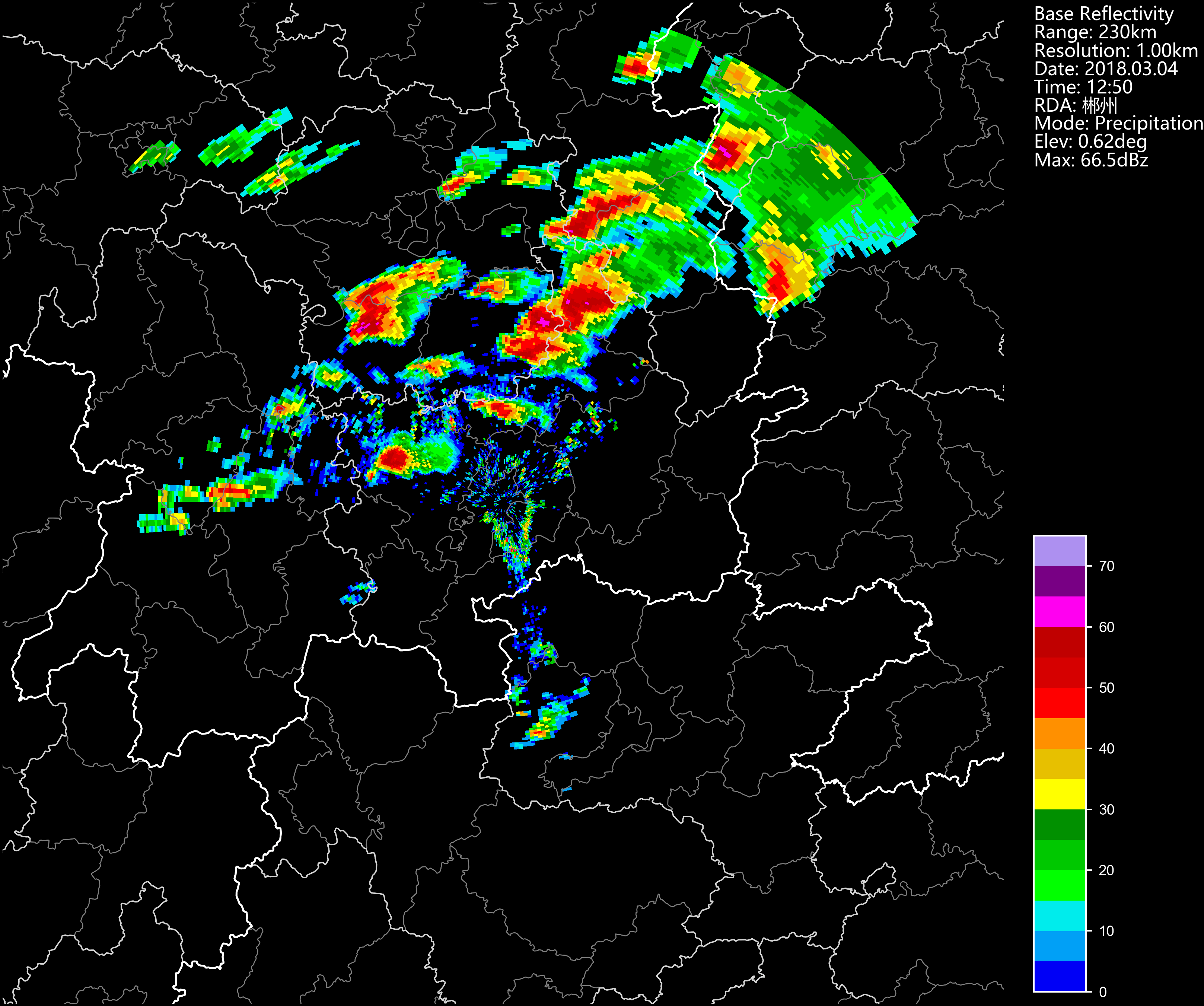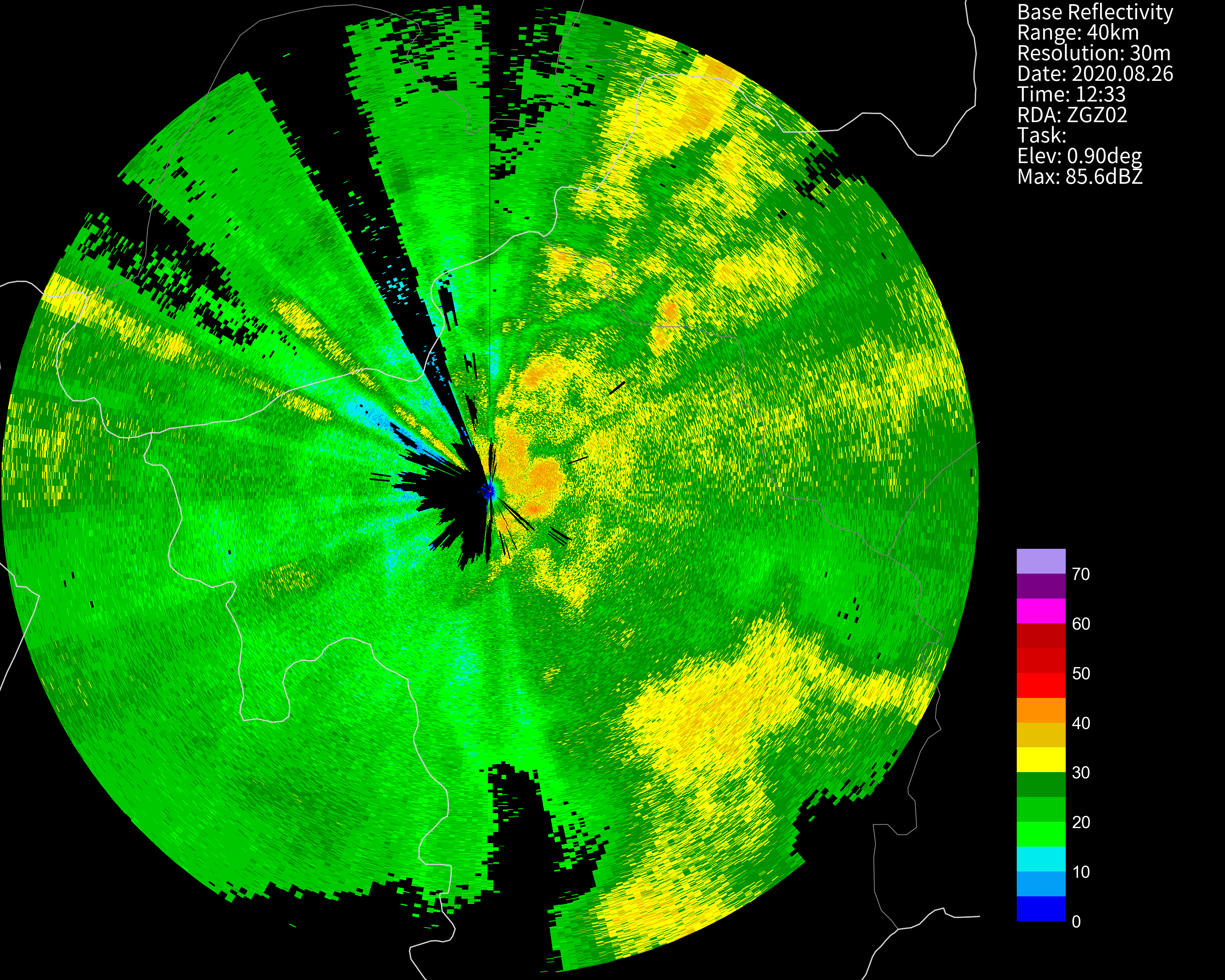#### PPI reflectivity combined with cross-section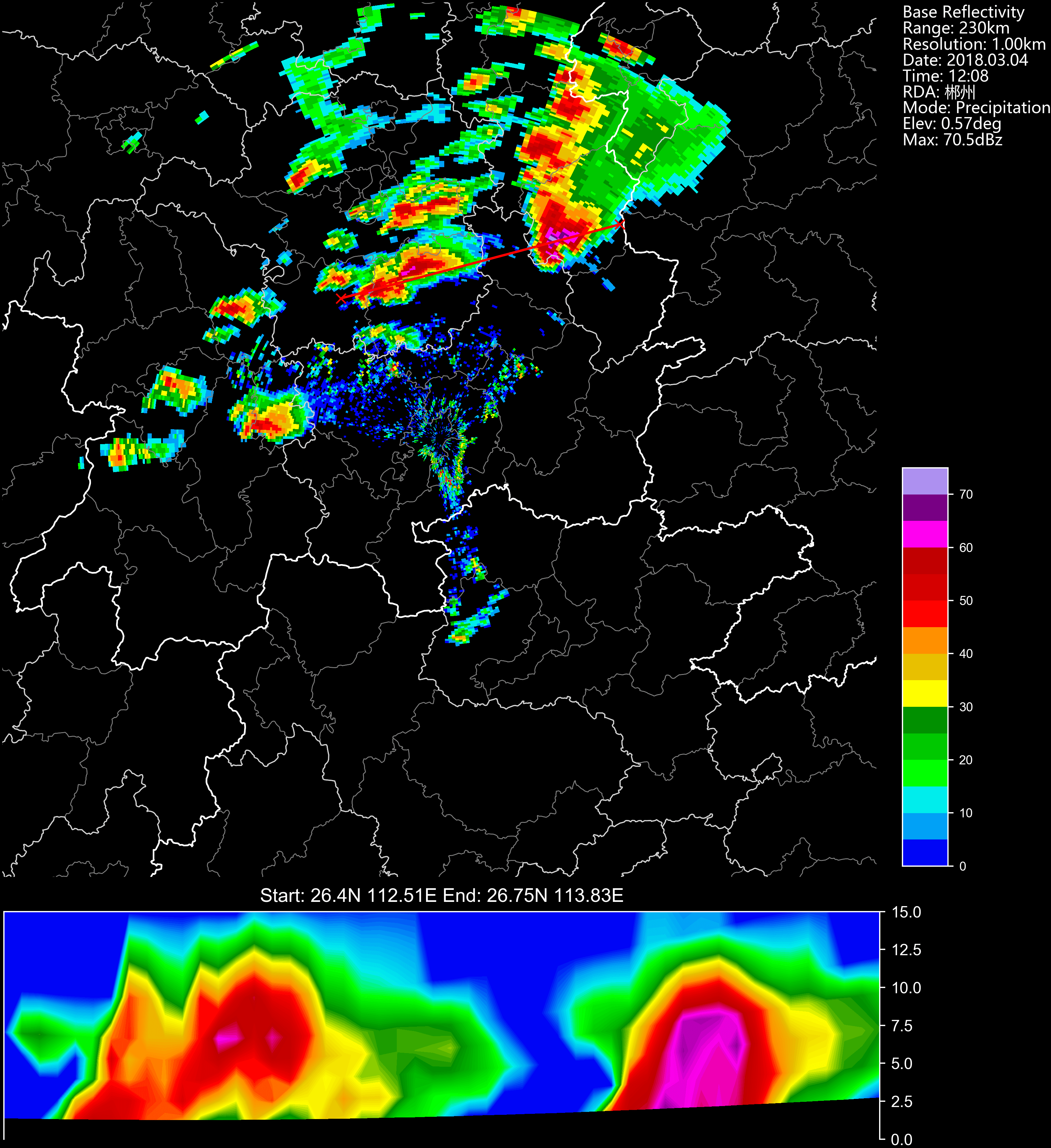#### Cross-section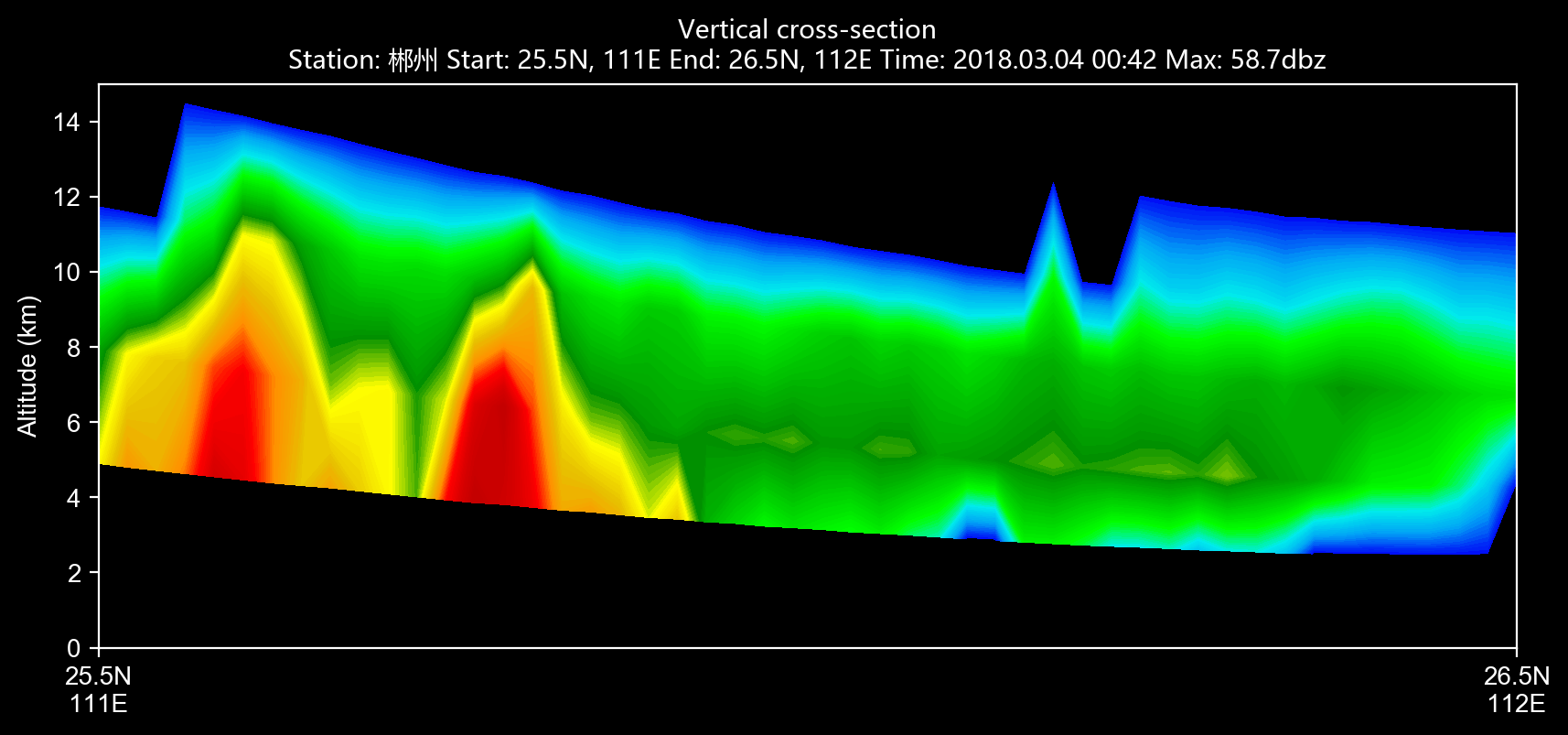#### Cross-section other than reflectivity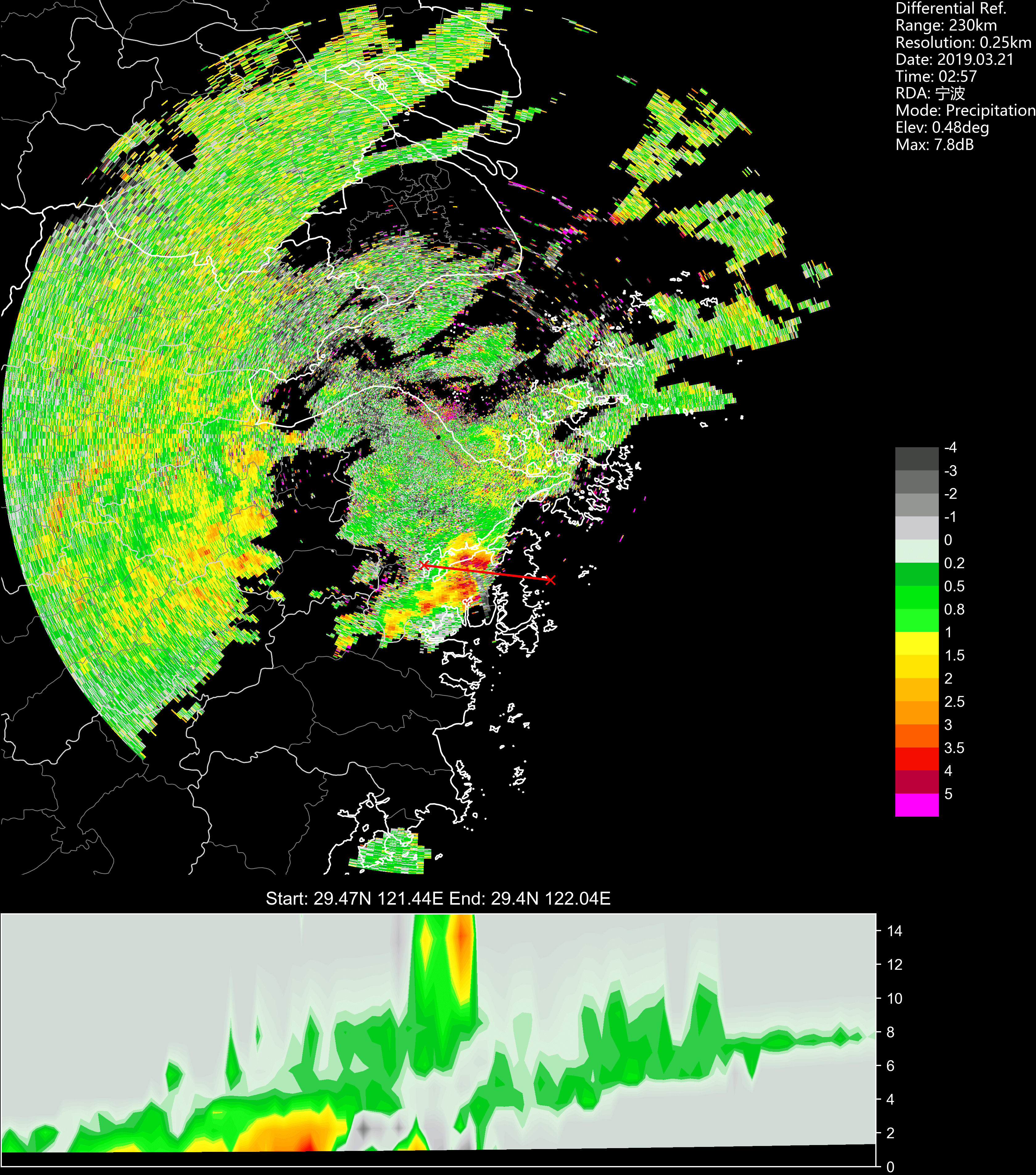#### RHI reflectivity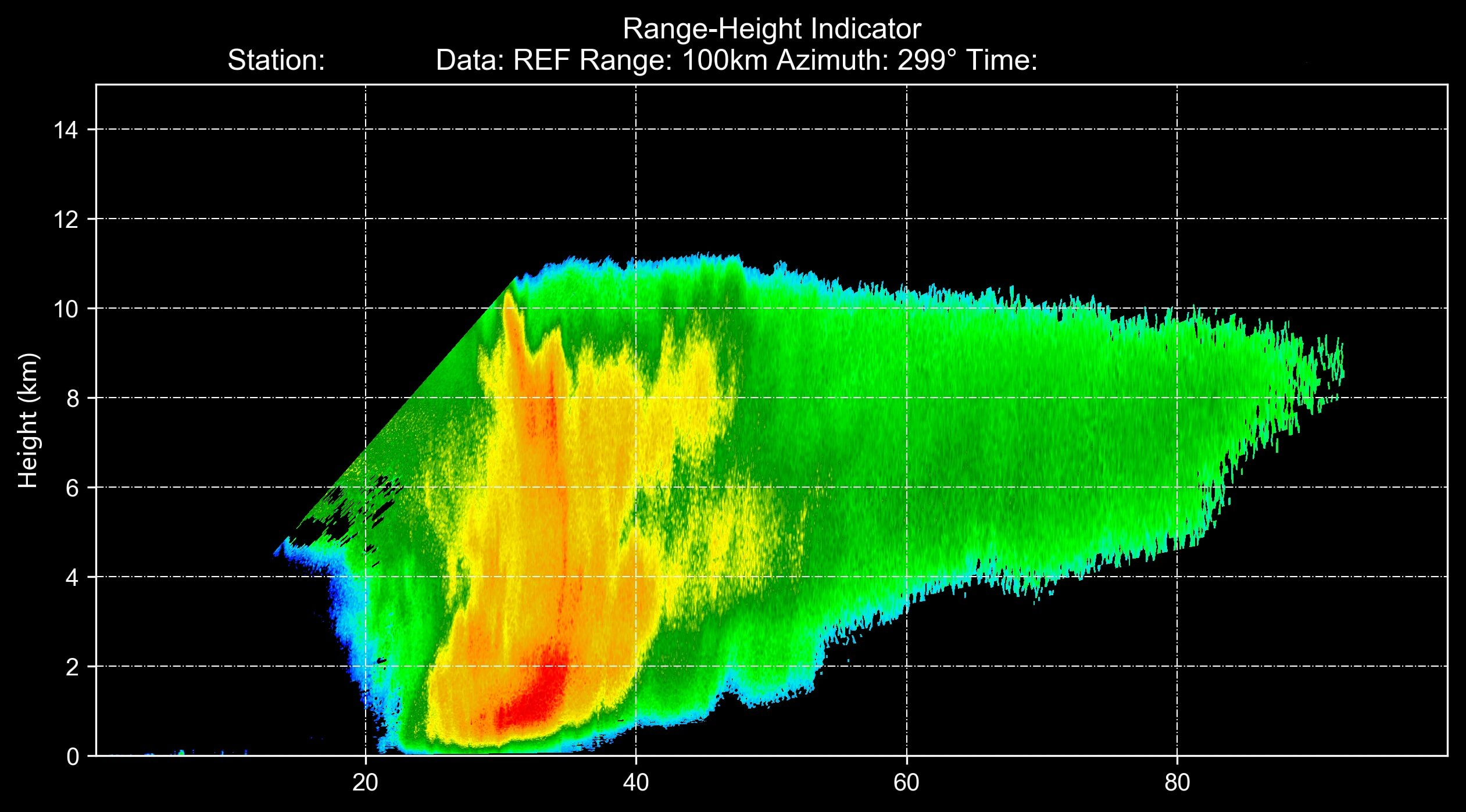## Citation## Papers that use plots generated by `PyCINRAD`

1. Recognition and Analysis of Biological Echo Using WSR-88D Dual-polarization Weather Radar in Nanhui of Shanghai doi: 10.16765/j.cnki.1673-7148.2019.03.015

## Notes

The hydrometeor classfication algorithm comes from Dolan, B., S. A. Rutledge, S. Lim, V. Chandrasekar, and M. Thurai, 2013: A Robust C-Band Hydrometeor Identification Algorithm and Application to a Long-Term Polarimetric Radar Dataset. J. Appl. Meteor. Climatol., 52, 2162–2186, https://doi.org/10.1175/JAMC-D-12-0275.1.

If you are interested in this program, you can join the developers of this program. Any contribution is appreciated!